# C – File I/O

In this article, we will learn how to operate over files using a C program. A single C file can read, write, move, and create files in our computer easily using a few functions and elements included in the C File I/O system. We can easily manipulate data in a file regardless of whether the file is a text file or a binary file using functions like fopen(), fclose(), fprintf(), fscanf(), getc(), putc(), getw(), fseek(), etc.

## What are files in C?

A file is used to store huge data. C provides multiple file management functions like file creation, opening and reading files, Writing to the file, and closing a file. The file is used to store relevant data and file handling in C is used to manipulate the data.

## Types of Files in C

There are mainly two types of files that can be handled using File Handling in C as mentioned below:

1. Text Files
2. Binary Files

### 1. Text Files

These are simple text files that are saved by the (.txt) extension and can be created or modified by any text editor. Text file stores data in the form of ASCII characters and is used to store a stream of characters.

### 2. Binary Files

It is stored in binary format instead of ASCII characters. Binary files are normally used to store numeric Information (int, float, double). Here data is stored in binary form i.e, (0’s and 1’s).

## C Files Operations

C Files can perform multiple useful operations that are mentioned below:

1. Creation of a new file (fopen with attributes as “a” or “a+” or “w” or “w+”).
2. Opening an existing file (fopenopen).
3. Reading from file (fscanf or fgets).
4. Writing to a file with fprintf or fputs.
5. Moving file pointer associated with a given file to a specific position. (fseek, rewind).
6. Closing a file (close).

## File Pointer declaration

For performing operations on the file, a special pointer called File pointer is used that can be declared as:

`FILE file_ptr;`

We can open the file as

`file_ptr = fopen("fileName.txt", "w");`

## File Modes in C

The second parameter i.e, “w” can be changed according to the table below:

If you want to handle binary files then access modes like “rb”, “wb”, “ab”, “rb+”, r+b”, “wb+”, “w+b”, “ab+”, “a+b” will be used mostly.

## Opening a file in C

To perform the opening and creation of a file in c we can use the fopen() function which comes under stdio.h header file.

Syntax:

`p = fopen("fileopen", "mode");`

Example:

`p = fopen("Hello.txt", r);`

## Creating a File in C

As now we know how to open a file using fopen() now the question arises about creation. The creation of a file is as simple as opening a file. As, if the while opening a file for writing or appending is done with either write(“w”) or append(“a”) mode then in the case where the file doesn’t exist a new file is created.

Example:

## C

 `// C program to Create a file ` `#include ` `#include ` ` `  `// Driver code ` `int` `main() ` `{ ` `    ``// File Pointer declared ` `    ``FILE``* ptr; ` ` `  `      ``// File opened ` `    ``ptr = ``fopen``(``"./Hello.txt"``, ``"w"``); ` ` `  `      ``// Failed Condition ` `    ``if` `(ptr == NULL) { ` `        ``printf``(``"Error Occurred While creating a "` `               ``"file !"``); ` `        ``exit``(1); ` `    ``} ` ` `  `      ``// File closed ` `    ``fclose``(ptr); ` ` `  `      ``// Data is finally Inserted ` `    ``printf``(``"File created\n\n"``); ` ` `  `    ``return` `0; ` `} `

Output: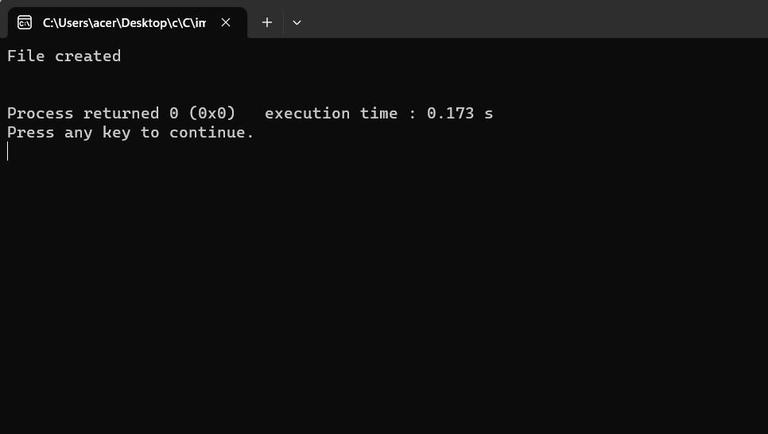The output of the Creating a File in C

File Created: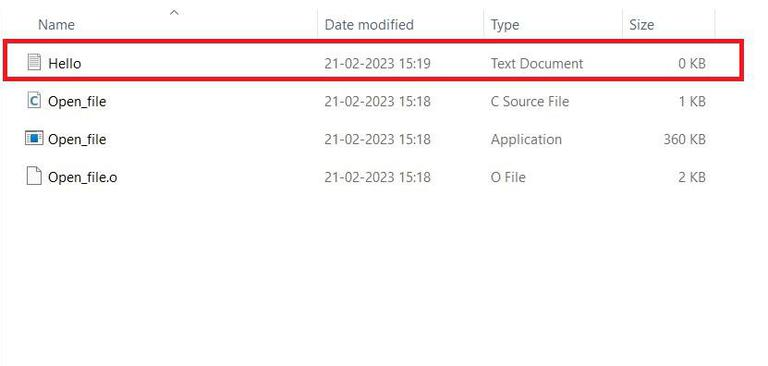Hello.txt file created using the C program

## Writing a File in C

### 1. Writing to a text file in C

fprintf() and fscanf() are used to read and write in a text file in C programming. They expect a pointer to the structure FILE since they are file versions of print() and scanf().

## C

 `// C program to write contents ` `// to the file ` `#include ` `#include ` ` `  `// Driver code ` `int` `main() ` `{ ` `    ``// File Pointer declared ` `    ``FILE``* ptr; ` ` `  `      ``// File opened ` `    ``ptr = ``fopen``(``"./Hello.txt"``, ``"w+"``); ` ` `  `      ``// Failed Condition ` `    ``if` `(ptr == NULL) { ` `        ``printf``(``"Error Occurred While writing to a text "` `               ``"file !"``); ` `        ``exit``(1); ` `    ``} ` ` `  `      ``// Data to be inserted ` `    ``char` `str[] = ``"This is all the Data to be inserted in "` `                 ``"File by GFG."``; ` ` `  `      ``// Puts data inside the file ` `    ``fputs``(str, ptr); ` `   `  `      ``// File closed ` `    ``fclose``(ptr); ` ` `  `      ``// Data is finally Inserted ` `    ``printf``(``"Data Written Inside the file\n\n"``); ` ` `  `    ``return` `0; ` `}`

Output: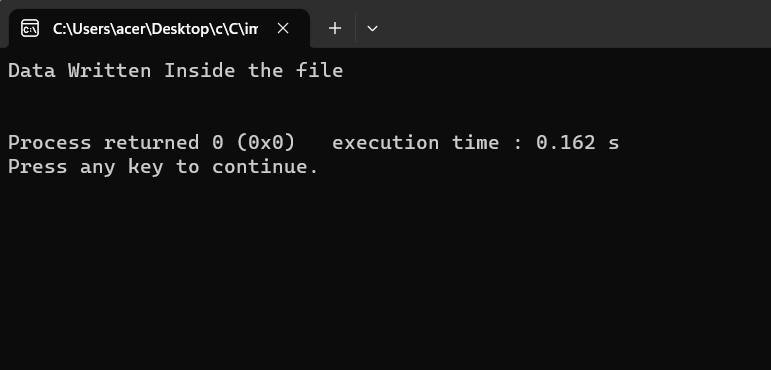The output of Writing to a text file in C

File Created: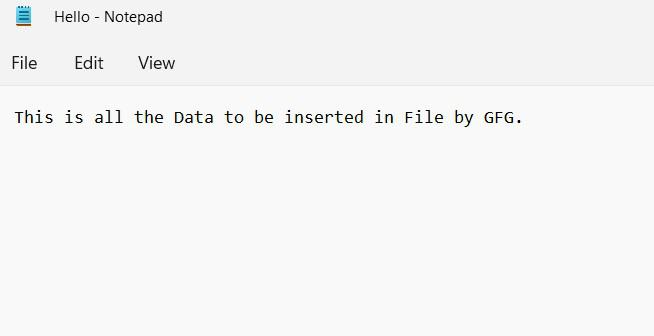Writing to a text file in C

The above program takes a string from a user and stores it in text_file.text.After compiling this program a text file named temp_text.txt will be created in the C_Program folder. Inside the file, we can see the string that we entered.

### 2. Writing to a Binary File

fwrite() function takes four arguments address of data, size of the data which is to be written on the disk, number of data types, and a pointer to the file where we want to write.

Syntax:

`fwrite(const void *ptr,size_of_elements,number_of_elements, FILE *a_file);`

Below is the C program to write to a binary file:

## C

 `// C program to write to a ` `// binary file ` `#include ` `#include ` ` `  `// Struct declared ` `struct` `Num { ` `    ``int` `n1, n2; ` `}; ` ` `  `// Driver code ` `int` `main() ` `{ ` `      ``// variables declared ` `    ``int` `n; ` `    ``struct` `Num obj; ` `   `  `      ``// File Pointers declared ` `    ``FILE``* fptr; ` `   `  `      ``// Failure Condition ` `    ``if` `((fptr = ``fopen``(``"temp.bin"``, ``"wb"``)) == NULL) { ` `        ``printf``(``"Error! opening file"``); ` ` `  `        ``// if file pointer returns NULL program ` `        ``// will exit ` `        ``exit``(1); ` `    ``} ` ` `  `       `  `    ``for` `(n = 1; n < 10; n++) { ` `        ``obj.n1 = n; ` `        ``obj.n2 = 12 + n; ` `       `  `      ``// Data written  ` `        ``fwrite``(&obj, ``sizeof``(``struct` `Num), 1, fptr); ` `    ``} ` ` `  `      ``// File closed ` `    ``fclose``(fptr); ` ` `  `    ``printf``(``"Data in written in Binary File\n\n"``); ` ` `  `    ``return` `0; ` `}`

Output: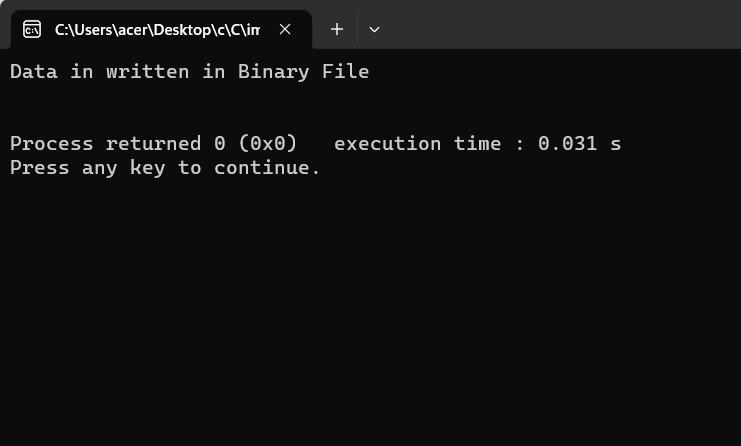The output of Writing to a Binary File

Image of created file: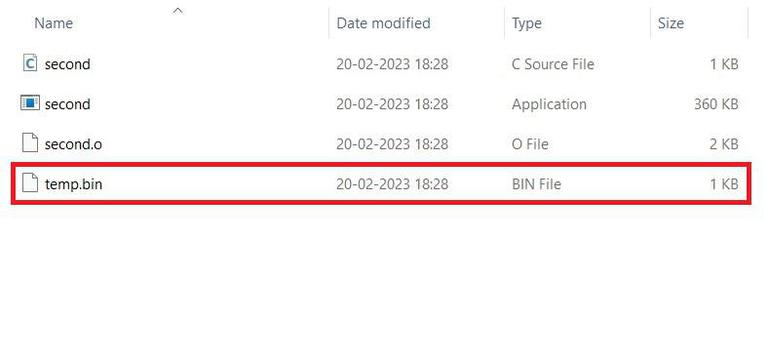.bin file created with the data

Note: fread() is used to read from a binary file and fwrite() is used to write to a file on the disk.

### 1. Reading text files in C

Below is the C program to read the contents from the file:

## C

 `// C program to read contents ` `// from the file ` `#include ` `#include ` ` `  `// Driver code ` `int` `main() ` `{ ` `  ``char` `str; ` `  ``FILE``* ptr; ` ` `  `  ``ptr = ``fopen``(``"Hello.txt"``, ``"r"``); ` ` `  `  ``if` `(ptr == NULL)  ` `  ``{ ` `    ``printf``(``"Error While opening file"``); ` `         `  `    ``// if the pointer returns NULL  ` `    ``// program will exit ` `    ``exit``(1); ` `  ``} ` `   `  `  ``if``(``fgets``(str, 80, ptr) != NULL) ` `  ``{ ` `    ``puts``(str); ` `  ``} ` `  ``fclose``(ptr); ` ` `  `  ``return` `0; ` `}`

Output: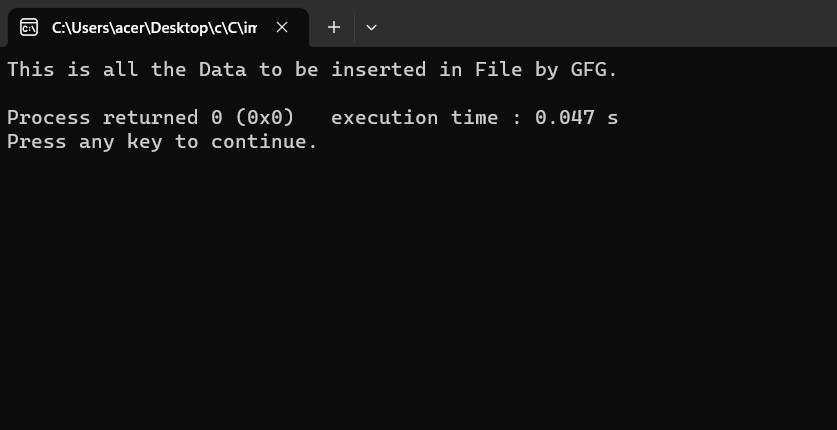The output of Reading text files in C

### 2. Reading from a Binary File

fread() function also takes four arguments that are similar to fwrite() function in C Programming.

Syntax:

`fwrite(const void *ptr,size_of_elements,number_of_elements, FILE *a_file);`

Below is the C program to read from a binary file:

## C

 `// C Program to Read from a  ` `// binary file using fread() ` `#include ` `#include ` `struct` `Num  ` `{ ` `    ``int` `n1, n2; ` `}; ` ` `  `// Driver code ` `int` `main() ` `{ ` `  ``int` `n; ` `  ``struct` `Num obj; ` `  ``FILE``* fptr; ` `  ``if` `((fptr = ``fopen``(``"temp.bin"``, ``"rb"``)) == NULL)  ` `  ``{ ` `    ``printf``(``"Error! opening file"``); ` `         `  `    ``// If file pointer will return NULL ` `    ``// Program will exit. ` `    ``exit``(1); ` `  ``} ` `     `  `  ``// else it will return a pointer  ` `  ``// to the file. ` `  ``for` `(n = 1; n < 10; ++n)  ` `  ``{ ` `    ``fread``(&obj, ``sizeof``(``struct` `Num), 1, fptr); ` `    ``printf``(``"n1: %d\tn2: %d\n"``, obj.n1, obj.n2); ` `  ``} ` `   `  `  ``fclose``(fptr); ` `  ``return` `0; ` `}`

Output: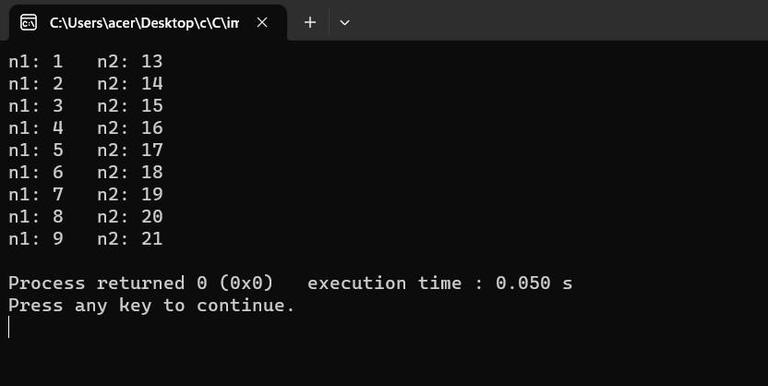The output of Reading from a Binary File

Explanation: In the above program, we have read the same file GFG.bin and are looping through records one by one. We read a single Num record of Num size from the file pointed by *fptr into the structure Num. We’ll get the same record that we inserted in the previous program.

## Moving File Pointers to Specific Positions

fseek() and rewind() are the two methods in C programming that can be used to move the file pointer. Let us check both methods:

### 1. fseek() in C Programming

fseek() function is used to set the file pointer to the specified offset and write data into the file.

Syntax:

`int fseek(FILE *stream, long int offset, int whence);`

Here,

• whence can be SEEK_SET, SEEK_CUR and SEEK_END.
• SEEK_END: It denotes the end of the file.
• SEEK_SET: It denotes starting of the file.
• SEEK_CUR: It denotes the file pointer’s current position.

Below is the C program to implement fseek():

## C

 `// C program to implement ` `// fseek ` `#include ` `#include ` ` `  `// Driver code ` `int` `main() ` `{ ` `      ``// string declared ` `    ``char` `str; ` `   `  `      ``// File Pointer declared ` `    ``FILE``* ptr; ` ` `  `      ``// File Opened ` `    ``ptr = ``fopen``(``"Hello.txt"``, ``"w+"``); ` `     `  `      ``// Puts data in File ` `      ``fputs``(``"Welcome to GeeksforGeeks"``, ptr); ` ` `  `      ``// fseek function used ` `    ``fseek``(ptr, 11, SEEK_SET); ` `   `  `      ``// puts programming in place of at position defined  ` `      ``// After 11 elements ` `    ``fputs``(``"Programming  "``, ptr); ` `    ``fclose``(ptr); ` ` `  `      ``// Reading the file ` `    ``ptr = ``fopen``(``"Hello.txt"``, ``"r+"``); ` `    ``if` `(``fgets``(str, 80, ptr) != NULL) { ` `        ``puts``(str); ` `    ``} ` ` `  `      ``// Close the opened file ` `    ``fclose``(ptr); ` `   `  `    ``return` `0; ` `}`

Output: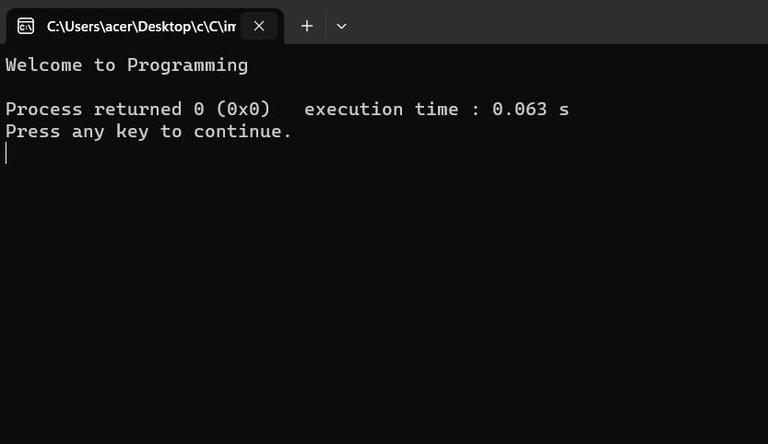The output of  fseek() in C

Image of the File: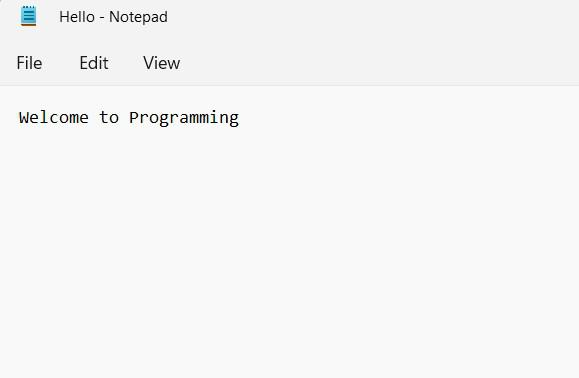Changes in the file

### 2. rewind() in C

rewind() function sets the file pointer to the beginning of the file.

Syntax:

`void rewind(FILE *stream);`

Below is the C Program to implement rewind():

## C

 `// C program to implement ` `// rewind() ` `#include ` `#include ` ` `  `// Driver code ` `int` `main() ` `{ ` `  ``// String declared ` `    ``char` `str; ` `   `  `      ``// File Pointer declared ` `    ``FILE``* ptr; ` ` `  `      ``// File opened ` `    ``ptr = ``fopen``(``"Hello.txt"``, ``"w+"``); ` `   `  `      ``// Puts data inside the file ` `    ``fputs``(``"Welcome to GeeksforGeeks"``, ptr); ` ` `  `      ``// File closed ` `    ``fclose``(ptr); ` ` `  `      ``// File open to read ` `    ``ptr = ``fopen``(``"Hello.txt"``, ``"r+"``); ` `    ``if` `(``fgets``(str, 200, ptr) != NULL) { ` `        ``puts``(str); ` `    ``} ` `   `  `      ``// Rewind function called ` `      ``// Sets ptr to beginning ` `    ``rewind``(ptr); ` `    ``if` `(``fgets``(str, 200, ptr) != NULL) { ` `        ``puts``(str); ` `    ``} ` ` `  `    ``fclose``(ptr); ` `    ``return` `0; ` `}`

Output: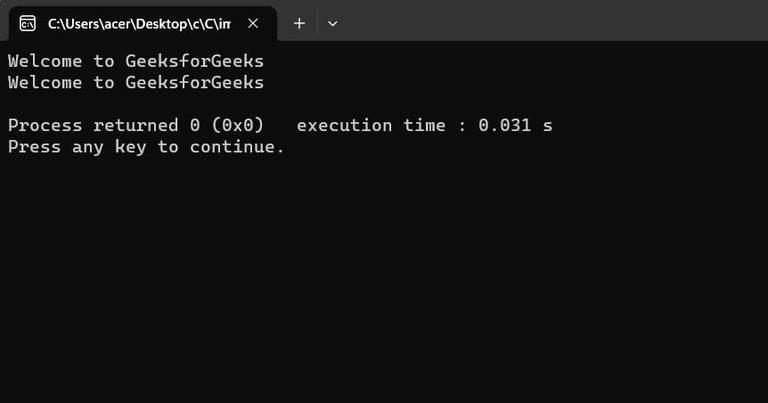The output of rewind() in C

Image of the File: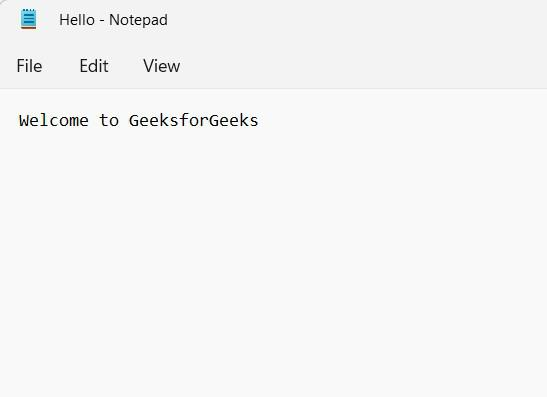File after running the program

## FAQs on C File I/O

### 1. How to open a file in C?

File in C can be opened using file pointer and fopen function. In the first step, we need to declare the file pointer and in the second step we can use fopen with the name of the file to be opened and the mode(read, write, append) for using the file.

Example:

```FILE file_ptr;
file_ptr = fopen("fileName.txt", "w");```

### 2. How to create a file in C?

The file can be created using two modes: append and write. We need to simply open the file with either mode to create the file.

### 3. How to read a file in C?

File in C can be read by opening the file in read mode. And we can get strings from the file using the fgets function.

### 4. How to write to a file in C?

File in C can be read by opening the file in read mode. And we can get strings from the file using the fputs function.

### 5. What is the use of fseek() in C?

fseek() is used for moving file pointer associated with a given file to a specific position in a file.

### 6. What are modes in C file io?

Modes in C file IO defines the functionality of the file pointer,i.e. means whether it can read, write, append, etc in a file.

### 7. What are different operations which can be performed on a file in C?

There are multiple operations that can be performed in a file which are creating, opening, reading, writing, and moving.

Whether you're preparing for your first job interview or aiming to upskill in this ever-evolving tech landscape, GeeksforGeeks Courses are your key to success. We provide top-quality content at affordable prices, all geared towards accelerating your growth in a time-bound manner. Join the millions we've already empowered, and we're here to do the same for you. Don't miss out - check it out now!

Previous
Next